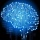Home Communities
IT Knowledge
Inspiration
Languages
EN

# JavaScript - generate unique id (useful in web browser)

2 points
Created by:Root-ssh
151380

In this short article, we would like to show how to generate a unique id that can be used on UI.

The below solution to provide unique IDs that is created using incremented counter + current time + random number.

The idea bases on:

• incremented counter is always unique per loaded webpage  - refresh / reload causes reset,
• current time provides unique time between webpage reloading / refreshing - only incorrect time in the operating system can duplicate values,
• random number increases the probability that generated ID will be unique.

Note: the way how to generate UUID was described in this article.

Advantages: the presented solution has very good performance - better than UUID implementation presented in this article.

Quick solution:

``````// ONLINE-RUNNER:browser;

function randimizeTime() {
return String(Date.now());
}

function randomizeNumbers() {
var text = String(Math.random());
for (var i = text.length; i < 19; ++i) {
text += '0';
}
return text.substring(2, 19);
}

var Generator = new function() {
var counter = 0;
var incrementCounter = function() {
return String(++counter);
};
this.generateId = function() {
var value = String(Math.random());
return incrementCounter() + '-' + randimizeTime() + '-' + randomizeNumbers();
};
};

// Usage example:

console.log(Generator.generateId());
console.log(Generator.generateId());
console.log(Generator.generateId());
console.log(Generator.generateId());
console.log(Generator.generateId());
console.log(Generator.generateId());``````

## ES6+ example

``````// ONLINE-RUNNER:browser;

let counter = 0;

const incrementCounter = () => {
return String(++counter);
};

const randimizeTime = () => {
return String(Date.now());
};

const randomizeNumbers = () => {
let text = String(Math.random());
for (let i = text.length; i < 19; ++i) {
text += '0';
}
return text.substring(2, 19);
}

const generateId = () => {
return incrementCounter() + '-' + randimizeTime() + '-' + randomizeNumbers();
};

// Usage example:

console.log(generateId());
console.log(generateId());
console.log(generateId());
console.log(generateId());
console.log(generateId());
console.log(generateId());``````

1. JavaScript - UUID function in Vanilla JS

## Alternative titles

Join to our subscribers to be up to date with content, news and offers.